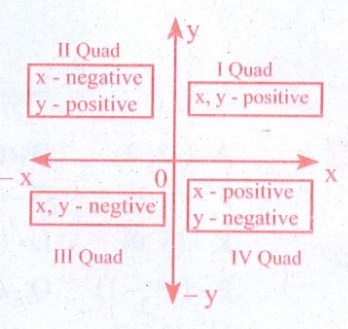Home | | Maths 8th Std | Exercise 3.8 (Graph Basics)

# Exercise 3.8 (Graph Basics)

8th Maths : Chapter 3 : Algebra : Graph : Exercise 3.8 : Text Book Back Exercises Questions with Answers, Solution

Exercise 3.8

Fill in the blanks:

(i) X- axis and Y-axis intersect at __________. [Answer: Origin (0, 0)]

(ii) The coordinates of the point in third quadrant are always__________. [Answer: negative]

(iii) (0, −5) point lies on ________axis. [Answer: Y−axis]

(iv) The x- coordinate is always ______on the y-axis. [Answer: zero]

(v) _________ coordinates are the same for a line parallel to Y-axis. [Answer: X]

2. Say True or False:

Solution:

(−10, 20)

x = − 10, y = 20

(− 10, 20) lies in second quadrant – True

(ii) (−9, 0) lies on the x-axis. [Answer: True]

Solution:

(−9, 0) on x − axis, Y− coordinate is always zero.

(−9, 0) lies on x axis – True

(iii) The coordinates of the origin are (1,1). [Answer: False]

Solution:

Coordinate of origin is (0, 0), not (1, 1). Hence – False

3. Find the quadrants without plotting the points on a graph sheet.

(3, -4), (5,7), (2,0), ( -3, -5), (4, -3), ( -7,2), ( -8,0), (0,10), ( -9,50).

Solution:If x & y coordinate are positive −1 quad

If x is positive, y is negative − IV quad

If x is negative, y is positive − II quad

If both are negative, then − III quad4. Plot the following points in a graph sheet.

A(5,2), B( -7, -3), C( -2,4), D( -1, -1), E(0, -5), F(2,0), G(7, -4), H( -4,0), I(2,3), J(8, -4), K(0,7).

Solution:5. Use the graph to determine the coordinates where each figure is located.a) Star (3, 2)

b) Bird (−2, 0)

c) Red Circle (−2, −2)

d) Diamond (−2, 2)

e) Triangle (−1, −1)

f) Ant (3, −1)

g) Mango (0, 2)

h) Housefly (2, 0)

i) Medal (−3, 3)

j) Spider (0, −2)

Exercise 3.8

1. (i) Origin (0,0) (ii) negative (iii) y-axis (iv) zero (v) x-Coordinate

2. (i) True (ii) True (iii) False

Tags : Questions with Answers, Solution | Algebra | Chapter 3 | 8th Maths , 8th Maths : Chapter 3 : Algebra
Study Material, Lecturing Notes, Assignment, Reference, Wiki description explanation, brief detail
8th Maths : Chapter 3 : Algebra : Exercise 3.8 (Graph Basics) | Questions with Answers, Solution | Algebra | Chapter 3 | 8th Maths